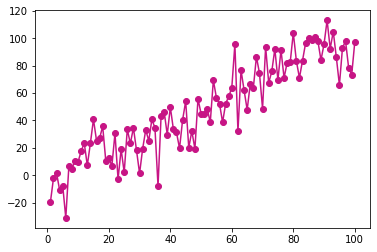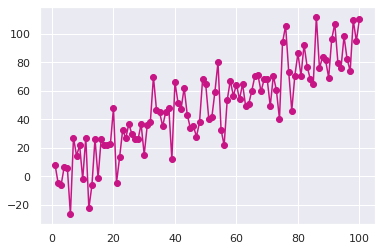Matplotlib allows you to make absolutely any type of chart. However the chart style of matplotlib library is not as fancy as seaborn style. It is possible to benefit from seaborn library style when plotting charts in matplotlib. You just need to load the seaborn library and use seaborn `set_theme()` function!

``````# library and dataset
from matplotlib import pyplot as plt
import pandas as pd
import numpy as np

# Create data
df=pd.DataFrame({'x_axis': range(1,101), 'y_axis': np.random.randn(100)*15+range(1,101), 'z': (np.random.randn(100)*15+range(1,101))*2 })

# plot with matplotlib
plt.plot( 'x_axis', 'y_axis', data=df, marker='o', color='mediumvioletred')
plt.show()````````````# library and dataset
from matplotlib import pyplot as plt
import pandas as pd
import numpy as np

# Create data
df=pd.DataFrame({'x_axis': range(1,101), 'y_axis': np.random.randn(100)*15+range(1,101), 'z': (np.random.randn(100)*15+range(1,101))*2 })

# Just load seaborn & set theme and the chart looks better:
import seaborn as sns
sns.set_theme()

# Plot
plt.plot( 'x_axis', 'y_axis', data=df, marker='o', color='mediumvioletred')
plt.show()

``````## Contact & Edit

👋 This document is a work by Yan Holtz. You can contribute on github, send me a feedback on twitter or subscribe to the newsletter to know when new examples are published! 🔥